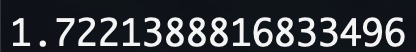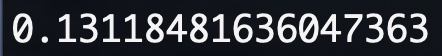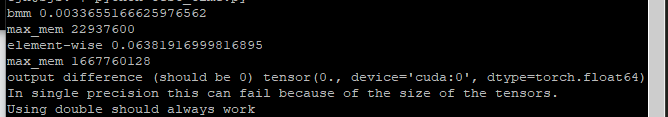# How to accelerate matrix/tensor multiplication/subtraction

hi,
I have two tensor a, b with the shape (batch_size,seq_len,dim)

the first operation is M=torch.bmm(a,b.transpose(1,2)) it works pretty fast.

and the second operation output the same result, but works pretty slowly:
a=a.unsqueeze(2)
b=b.unsqueeze(1)
N=(a*b).sum(-1)

my question is why does bmm work so fast , is it because the cuda optimize for matrix multiplication?
and the second question is is there accelerated way to do subtraction operation like bmm? the subtraction operation is like below:
a=a.unsqueeze(2)
b=b.unsqueeze(1)
N=(a-b).sum(-1)

Thank you!

Hi,

The difference shouldn’t be that big.
How do you measure the timing? Do you use `torch.cuda.synchronize()` properly when doing timing?

Actually,I found the big speed difference when running on GPU, and then I tested it on CPU, I measured timing like that:

I am not sure if this method is right.
Now I tested it again. the one without bmm is:and with bmm:So, can you tell what makes that big difference?

The CUDA api is asynchronous. So you only measure the time to launch the cuda kernel, not how long it takes to run it.
Here is a small benchmark code. It will show you the runtime and the peak memory usage for both cases.

Note that for such big number, the `float` overflows and you may want to use `double`.

``````import torch
import time

a = torch.randint(high=1000, size=(20, 200, 256)).float().cuda()
b = torch.randint(high=1000, size=(20, 200, 256)).float().cuda()

torch.cuda.synchronize()
start = time.time()

M = torch.bmm(a, b.transpose(1, 2))

torch.cuda.synchronize()
end = time.time()

print("bmm", end - start)
print("max_mem", torch.cuda.max_memory_allocated())

torch.cuda.synchronize()
start = time.time()

local_a = a.unsqueeze(2)
local_b = b.unsqueeze(1)
N = (local_a*local_b).sum(-1)

torch.cuda.synchronize()
end = time.time()

print("element-wise", end - start)
print("max_mem", torch.cuda.max_memory_allocated())

print("output difference (should be 0)", (N - M).abs().max())
print("In single precision this can fail because of the size of the tensors.")
print("Using double should always work")
``````

Really appreciate your reply and the code! I changed float to double(because float failed like you stated) and ran the code on GPU.
I got the right answer.However, the max_mem and time consumed between bmm and element-wise are of great difference:

similar issue occured when running on CPU.And now I am really confused. I assumed there is some optimization specifically for bmm, maybe parallelism?

The bmm use much less memory because it does not have to create the matrix `(a*b)` (after the unsqueeze). This matrix in the example is of size 20x200x200x256 and so use a HUGE amount of memory.
bmm is smarter in that it does not build this matrix but that means it cannot use of the parallelization power of the GPU as well.

But if bmm cannot use the parallelization power of GPU, why it showed up that it 's much faster than element-wise multiplication?because it does not build matrix explicitly? And if I want to do subtraction operation rather than multiplication , is there any way to make it faster like bmm?

That’s interesting on both Titan Black and Titan X, the bmm version is actually slower (4 to 10x). I guess that depend on your GPUI’m afraid at this level, the difference is mostly going to come from the parameters of how the job is launched on the GPU and the GPU itself.I tested it on TitanX but still got the same result:Maybe the cuda version matters? My cuda version is 10.

I use an old cuda 8.0.
Maybe they did big improvements in cuda10, that wouldn’t be surprisingok，thank you very much！